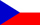Assoc. Prof. Petr Hasil, Ph.D.Conferences

• ICDDEA 2019: International Conference on Differential and Difference Equations Applications (Lisbon, Portugal)
přednáška: Oscillation behaviour of linear and half-linear equations with non-periodic coefficients
• NAAT 2018: International Conference on Numerical Analysis and Approximation Theory (Cluj-Napoca, Romania)
talk: Half-linear differential operators in oscillation theory
• ICDEA 2017: 23rd International Conference on Difference Equations and Applications (Timișoara, Romania)
talk: Conditional oscillation of second order linear and half-linear difference and differential equations
• Equadiff 15, 2015 (Lyon, France)
talk: Critical oscillation constants for half-linear differential and difference equations
• CDSDEA 2014: 10th AIMS Conference on Dynamical Systems, Differential Equations and Applications (Madrid, Spain)
talk: Critical oscillation constant for half-linear differential equations with coefficients having mean values
• CDDEA 2014: Conference on Differential and Difference Equations and Applications (Jasná, Slovakia)
talk: Oscillation constant for half-linear differential equations with coefficients having mean values
• Equadiff 13, 2013 (Praha, Czech Republic)
talk: Oscillation constant for half-linear equations with asymptotically almost periodic coefficients
• ICDEA 2013: 19th International Conference on Difference Equations and Applications (Muscat, Sultanate of Oman)
talk: Conditional oscillation of half-linear equations with asymptotically almost periodic coefficients
• CDDEA 2012: Conference on Differential and Difference Equations and Applications (Terchová, Slovakia)
talk: Conditional oscillation of Euler-type difference equation with almost periodic coefficients
• ICDEA 2011: 17th International Conference on Difference Equations and Applications (Trois-Rivières, Canada)
talk: Conjugacy criterion for self-adjoint difference equations
• ICDEA 2010: 16th International Conference on Difference Equations and Applications (Riga, Latvia)
talk: Disconjugacy of critical difference operators
• ICDSDEA 2010: 8th AIMS International Conference on Dynamical Systems, Differential Equations and Applications (Dresden, Germany)
talk: On criticality of higher order difference operators
• CDDEA 2010: Conference on Differential and Difference Equations and Applications (Rajecké Teplice, Slovakia)
talk: Critical difference operators
• CDEIT 2010: Colloquium on Differential Equations and Integration Theory (Křtiny, Czech Republic)
poster: Critical oscillation constant for half-linear differential equations with periodic coefficients
• ICDEA 2009: 15th International Conference on Difference Equations and Applications (Estoril, Portugal)
talk: Critical higher order Sturm-Liouville difference operators
• Equadiff 12, 2009 (Brno, Czech Republic)
talk: Critical higher order Sturm-Liouville difference operators
• CDDEA 2008: Conference on Differential and Difference Equations and Applications (Strečno, Slovakia)
talk: Conditional oscillation of half-linear differential equations with periodic coefficients

Lectures at:    Seminar on Differential Equations    Department of Mathematics and Statistics, Faculty of Science, Masaryk University

• 15.4.2019
Difference equations and their oscillation constants
• 10.10.2016
Conditional oscillation of differential equations
• 5.10.2015
Oscillation constant for differential and difference equations
• 29.4.2013
Oscillation of half-linear difference equations with asymptotically almost periodic coefficients
• 15.10.2012
Conditional oscillation of Euler-type difference equation with almost periodic coefficients
• 22.11.2010
Critical oscillation constant for half-linear differential equations with periodic coefficients
• 9.11.2009
Conjugacy of Sturm-Liouville difference equations of higher order
• 3.11.2008
Conditional oscillation of half-linear differential equations with periodic coefficients
Copyright © 2012 Petr Hasil   •   Design by Blondie (XHTML 1.0 Strict, CSS 2.1)